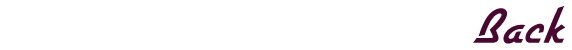### SWAT 2

```
Line of Sight Cheat:

Type in "los" (you may have to do it a few times)
when you're in a mission to toggle off the Line of
Sight. All characters will be visible, even if
they're not in any of your units' line of sight.
The problem is if you type it too slow, then your
terrorist guy surrenders. It also doesn't let you
perform any actions when the Line of Sight is off,
so you have to type "los" again if you want to
perform a action other than shooting. This is
especially fun to do when you're playing as the
terrorists. Even if all your guys are holed up in
one area, you can watch SWAT make entry and clear
the rooms.

All Mission Cheat:

To get all the missions, paste the following list
into the swat2.ini file in the swat2 root directory.
All missions can then be accessed through quick play.

[Missions Played]
SM1=1
SM2=1
SM3=1
SM4=1
SM5=1
SM6=1
SM7=1
SM8=1
SM9=1
SM10=1
SM11=1
SM12=1
SM13=1
SM14=1
SM15=1
TM16=1
TM17=1
TM18=1
TM19=1
TM20=1
TM21=1
TM22=1
TM23=1
TM24=1
TM25=1
TM26=1
TM27=1
TM28=1
TM29=1
TM30=1
```